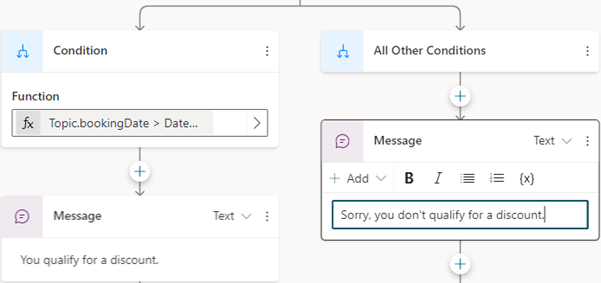# Create expressions using Power Fx

Power Fx is a low-code language that uses Excel-like formulas. Use Power Fx to create complex logic that allows your bots to manipulate data. For instance, a Power Fx formula can set the value of a variable, parse a string, or use an expression in a condition. For more information, see the Power Fx overview and formula reference.

Note

Power Fx formulas in Power Virtual Agents use US-style numbering. That is, the decimal separator is a period or dot, as in `12,567.892`. This means Power Fx parameters must be separated by commas (,).

## Use variables in a formula

To use a variable in a Power Fx formula, you must add a prefix to its name to indicate the variable's scope:

For example, to use the system variable `Conversation.Id` in a formula, you'd need to refer to it as `System.Conversation.Id`.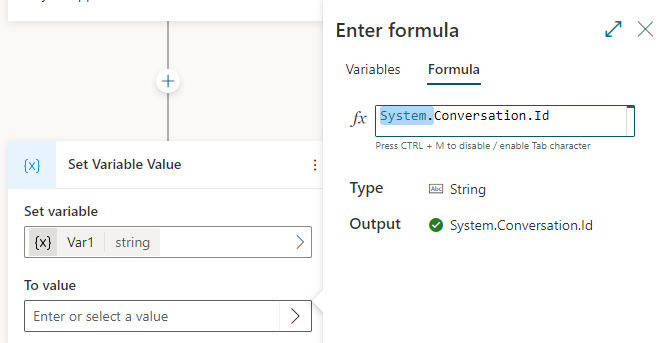## Use literal values in a formula

In addition to using variables in a Power Fx formula, you can enter literal values. To use a literal value in a formula, you must enter it in the format that corresponds to its type. The following table lists the data types and the format of their corresponding literal values.

Type Format examples
String `"hi"`, `"hello world!"`, `"chatbot"`
Boolean Only `true` or `false`
Number `1`, `532`, `5.258`, `-9201`
Table ``, `[45, 8, 2]`, `["cats", "dogs"]`
Record `{ id: 1 }`, `{ message: "hello" }`, `{ name: "John", info: { age: 25, weight: 175 } }`
DateTime `Time(5,0,23)`, `Date(2022,5,24)`, `DateTimeValue("May 10, 2022 5:00:00 PM")`
Choice Not supported
Blank Only `Blank()`

## Use Power Fx to set a variable

In this example, we'll use a Power Fx expression to store the customer's name and output it in capital letters.

1. Create a topic and add a Question node.

2. For Enter a message, enter `What is your name?`.

3. Under Identify, select the entity Person name.

4. Select the box under Save response as, and then select the variable `Var1` and name it `customerName`.5. Under the Question node, select + and then select Set a variable value.

6. Select the box under Set variable, and then select Create new and name it `capsName`.

7. In the To value box, select the > arrow, and then select the Formula tab.

8. In the fx box, enter `Upper(Text(Topic.customerName))`, and then select Insert.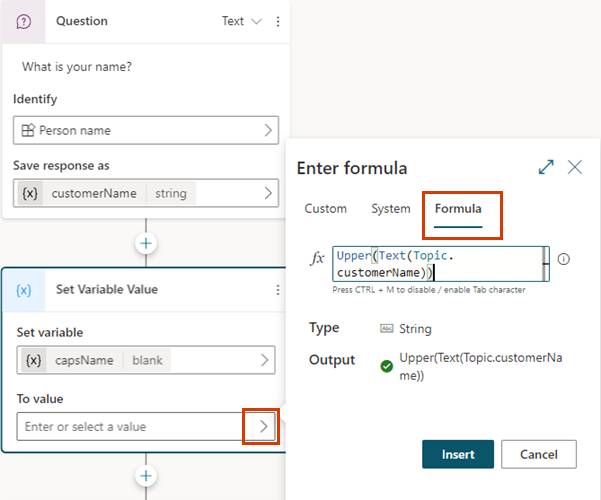9. Under the Question node, select + and then select Send a message.

10. Enter `HELLO `, select {x}, and then select `capsName`.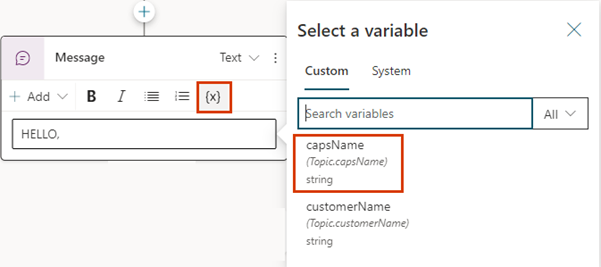## Use a Power Fx formula as a condition

To evaluate more complex expressions, set up Condition nodes to use Power Fx formulas.

In this example, the bot determines if a booking date qualifies for a discount. To do that, it checks whether the booking date provided by the customer is 14 days or more from the current date.

1. Create a topic and add a Question node.

2. For Enter a message, enter `Booking date?`.

3. Under Identify, select the entity Date and time.

4. Select the box under Save response as, and then select the variable `Var1` and name it `bookingDate`.5. Select the + icon and then select Add a condition.

6. In the Condition node, select the Node menu (), and then select Change to formula.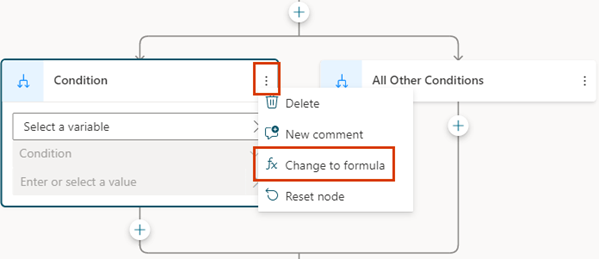7. In the Function box, select the > arrow, and then select the Formula tab.

8. Replace the contents of the fx box with the formula `Topic.bookingDate > (DateAdd (Now(), 14))`, and then select Insert.

9. Under the Condition node, add a Send a message node and enter the message `You qualify for a discount`.

10. Under the All Other Conditions node, add a Send a message node and enter the message, `Sorry, you don't qualify for a discount`.